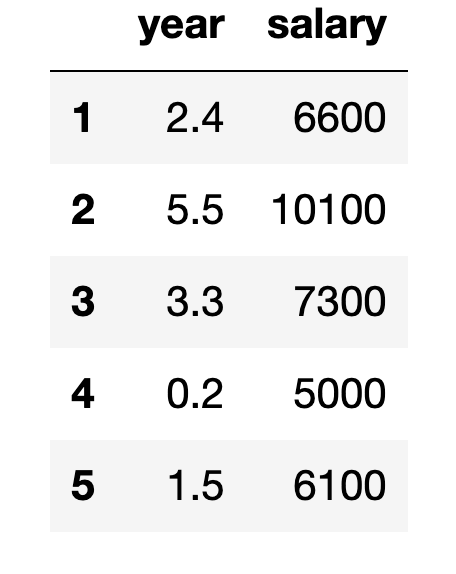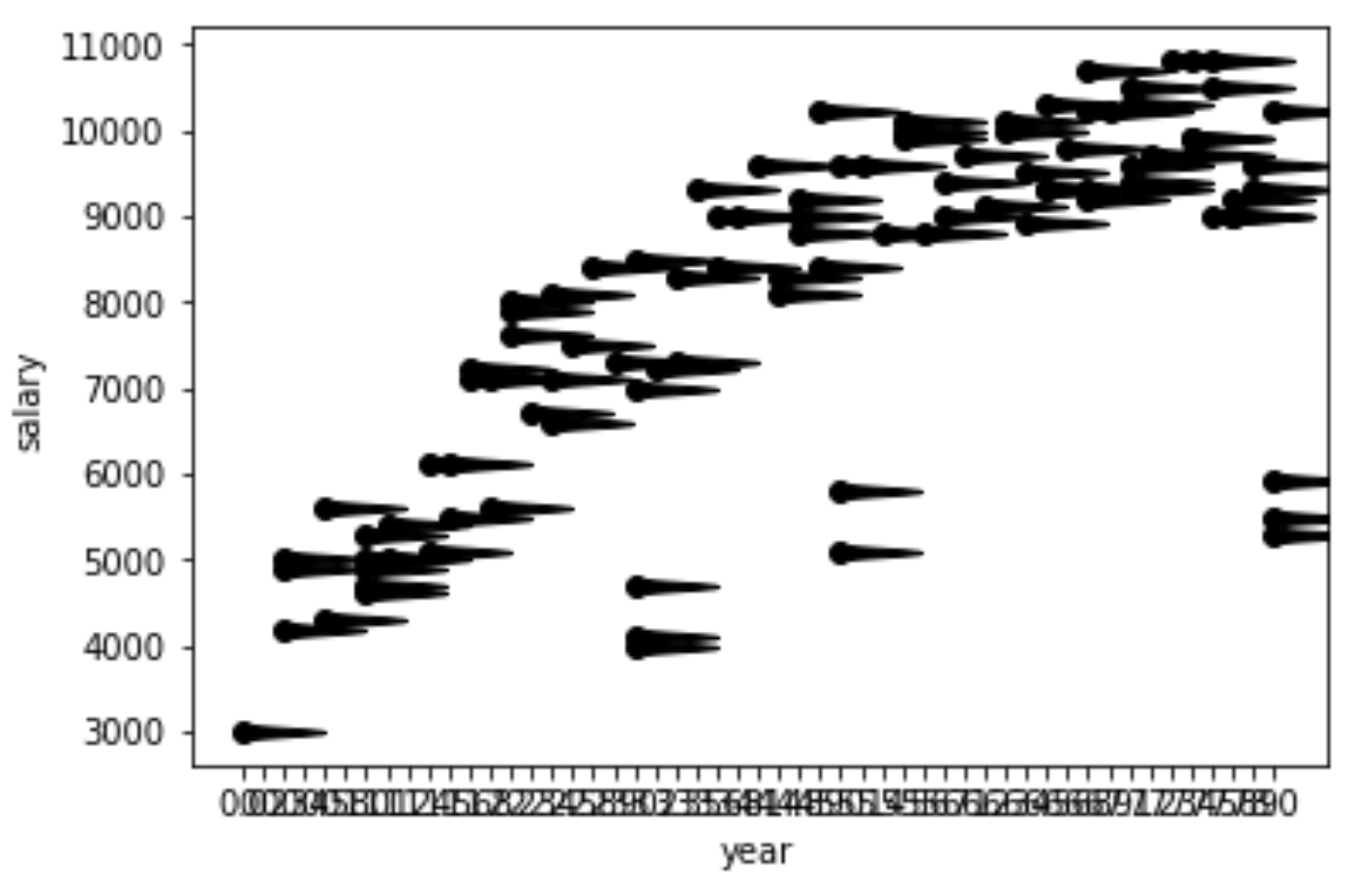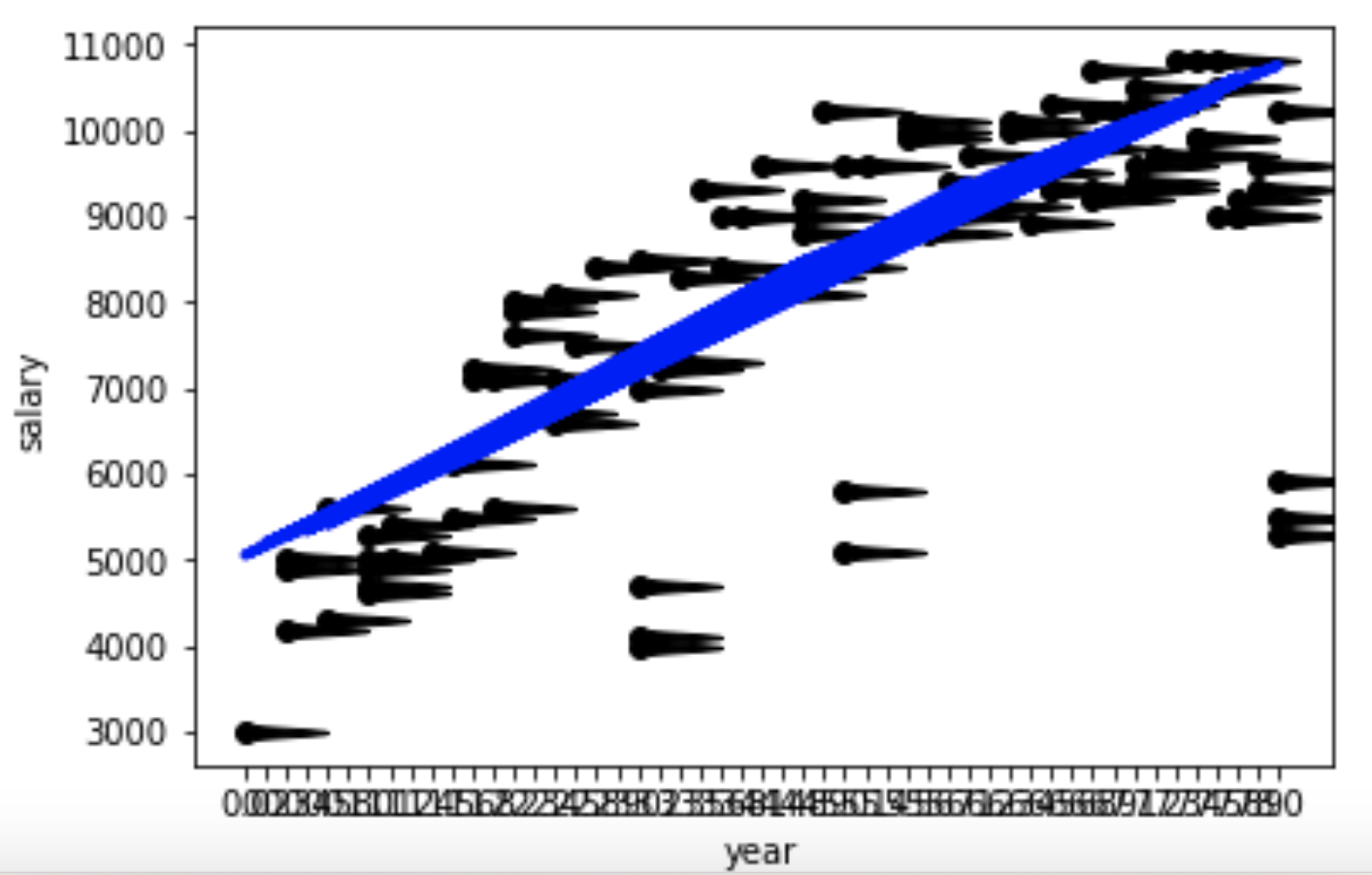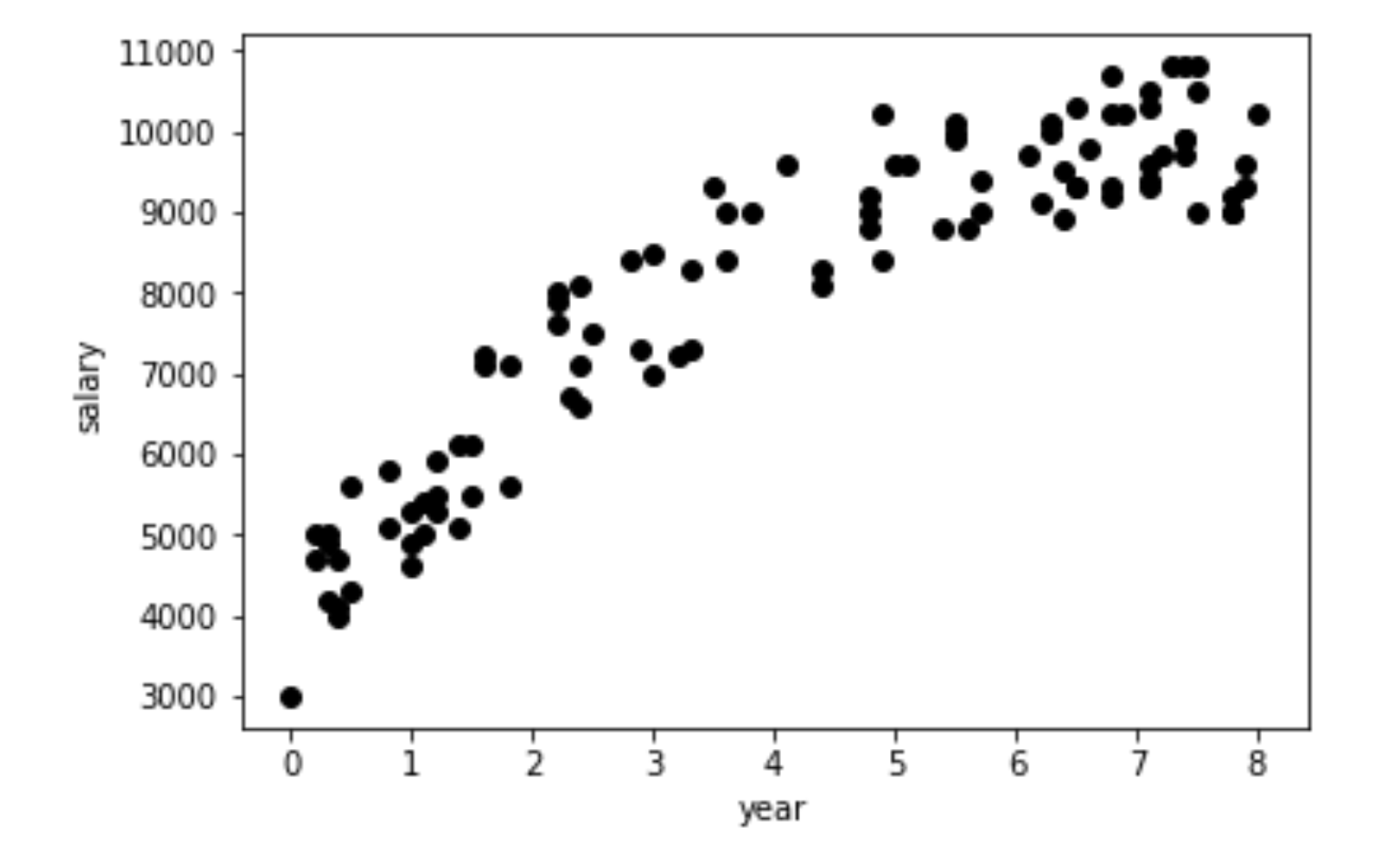matplotlib绘制散点图为什么画出来点变小蝌蚪了。如何解决？import pandas as pd
from matplotlib import pyplot as plt

df = pd.read_csv('data/salary.csv', index_col = 0)
X = df[['year']]
Y = df['salary'].values

plt.scatter(X,Y, color="black")
plt.xlabel('year')
plt.ylabel('salary')from sklearn.linear_model import LinearRegression
regr = LinearRegression()
regr.fit(X,Y)
plt.scatter(X,Y, color="black")
plt.plot(X, regr.predict(X), linewidth = 3, color = "blue")
plt.xlabel('year')
plt.ylabel('salary')2个回答

import matplotlib.pyplot as plt
df=df.sort_values(by=['year'])
X_plt = df['year'].values
Y_plt = df['salary'].values
X=df[['year']]
Y=df['salary']
plt.scatter(X_plt,Y_plt, color="black")
plt.xlabel('year')
plt.ylabel('salary')<div class="post-text" itemprop="text"> <p>如何更改用matplotlib绘制的图形的大小?</p> </div> <p>转载于:https://stackoverflow.com/questions/332289/how-do-you-change-the-size-of-figures-drawn-with-matplotlib</p>

python 散点图绘制坐标轴异常

matplotlib绘制直方图不显示横坐标值

 from matplotlib import pyplot as plt import matplotlib matplotlib.rcParams['font.sans-serif']=['SimHei'] # 用黑体显示中文 matplotlib.rcParams['axes.unicode_minus']=False # 正常显示负号 import numpy as np score_array = np.array(score_list) plt.hist(score_array, bins=10) plt.xlabel("区间") plt.ylabel("数量") plt.show()  ![图片说明](https://img-ask.csdn.net/upload/201910/15/1571106982_98227.png) 如图可见，直方图横坐标有一条黑线，百思不得其解，求大神解答，万分感谢

matplotlib所绘制的图像在全屏查看时比例失调，怎么解决？

python matplotlib.pyplot画折线图

Python画散点图，利用mpldatacursor为每个点作鼠标点击标记，但所有点标签显示都一样

matplotlib如何做分组条形图？？

![图片说明](https://img-ask.csdn.net/upload/201805/02/1525267299_368018.jpg) 如上图，要求有标题，横纵坐标都为字母，右边有热力图图例，总之越像越好。最好标题和横纵坐标都和上图一致，中间的数据以列表形式给出，代码中多加点注释，我好理解一点。本人新手，以前没接触过绘图，知道很麻烦各位大神，请大神见谅。谢谢！ 其实关于matplotlib生成热力图的问题我也看过不少，但好像没有完全能用的。我看到有个相似的代码如下，但缺少右边的colorbar，请问大神如何修改代码添加colorbar？： import matplotlib.pyplot as plt import numpy as np column_labels = list('ABCD') row_labels = list('WXYZ') data = np.random.rand(4,4) fig, ax = plt.subplots() heatmap = ax.pcolor(data, cmap=plt.cm.Blues) ax.set_xticks(np.arange(data.shape)+0.5, minor=False) ax.set_yticks(np.arange(data.shape)+0.5, minor=False) ax.invert_yaxis() ax.xaxis.tick_top() ax.set_xticklabels(row_labels, minor=False) ax.set_yticklabels(column_labels, minor=False) plt.show()

matplotlib绘制k线图如何跳过周末等数据空白日期，让图形连贯起来？

#!/usr/bin/python # -*- coding: UTF-8 -*- from pylab import * import talib from pylab import * import mpl_finance as mpf from matplotlib.pylab import date2num import pandas as pd import datetime mpl.rcParams['font.sans-serif'] = ['SimHei'] quotes = [] stock = pd.read_csv('99999.csv', index_col=None) for row in range(260): sdate_plt = row print(sdate_plt) sopen = stock.loc[row, 'open'] shigh = stock.loc[row, 'high'] slow = stock.loc[row, 'low'] sclose = stock.loc[row, 'close'] datas = (sdate_plt, sopen, shigh, slow, sclose) # 按照 candlestick_ohlc 要求的数据结构准备数据 quotes.append(datas) fig, ax = plt.subplots(facecolor=(0, 0.3, 0.5), figsize=(12, 8)) fig.subplots_adjust(bottom=0.1) ax.xaxis_date() plt.xticks(rotation=45) # 日期显示的旋转角度 plt.title('2018年7月1日至今k线图',fontsize=18) plt.ylabel('价格（元）',fontsize=15) mpf.candlestick_ohlc(ax, quotes, width=0.7, colorup='r', colordown='green') # 上涨为红色K线，下跌为绿色，K线宽度为0.7 plt.annotate(s='brown x = buying; green x = selling',xy=('2016-7-11',4400), xytext=('2016-7-11',4400),bbox=dict(boxstyle='round,pad=0.5', fc='yellow', ec='k', lw=2, alpha=0.5)) plt.grid(axis='y',linestyle='-.') plt.show() 1.  ![图片说明](https://img-ask.csdn.net/upload/201908/22/1566463928_276488.png)

Python/matplotlib：已知空间上一点坐标和长宽高值，如何画出立方体？

python使用matplotlib.pyplot绘制折线图纵坐标（y轴）与实际图内坐标不符，请问是什么原因呢？

python使用matplotlib.pyplot绘制折线图纵坐标（y轴）与实际图内坐标不符，效果图如下 （右下角是实际坐标） ![图片说明](https://img-ask.csdn.net/upload/202005/30/1590812415_307302.png) 绘图代码如下  plt.subplot(121),plt.plot([160,1600,3200,6400,9600,12800,16000],[85.66261,75.2519,72.2787,69.2498,67.4960,66.2274,65.2696],'r-*') plt.subplot(122),plt.plot([160,1600,3200,6400,9600,12800,16000],[0.99999980,0.99999443,0.99998277,0.99994450,0.99990344,0.99986035,0.99981919]) plt.show() `

python matplotlib 画两条折线图 其中一组数据有空值 如何处理？

python matplotlib 画两条折线图 其中一组数据有空值 如何处理？ import csv from datetime import datetime from matplotlib import pyplot as plt filename = 'data.csv' with open(filename) as f: reader = csv.reader(f) header_row = next(reader) dates = [] temp1 = [] temp2 = [] for row in reader: time = row + '-' + row + '-' + row current_date = datetime.strptime(time, "%Y-%m-%d") dates.append(current_date) tempm1 = int(float(row)) temp1.append(tempm1) tempm2 = int(row) temp2.append(tempm2) fig = plt.figure(dpi=128, figsize=(10,6)) plt.plot(dates, temp1, c='red') plt.plot(dates, temp2, c='blue') plt.show() 报错： C:\Users\yo\AppData\Local\Programs\Python\Python37\python.exe F:/论文/data/compare/compare.py Traceback (most recent call last): File "F:/论文/data/compare/compare.py", line 26, in <module> tempm2 = int(row) ValueError: invalid literal for int() with base 10: '20.075' Process finished with exit code 1 试过if 不为空值处理，然后数据对不齐了 我想要如果是空值图上就不显示 数据是有小数的所以就用int float 处理 请各位大神帮忙 谢谢！

2019 AI开发者大会

2019 AI开发者大会(AI ProCon 2019)是由中国IT社区CSDN主办的AI技术与产业年度盛会。多年经验淬炼，如今蓄势待发：2019年9月6-7日，大会将有近百位中美顶尖AI专家、知名企业代表以及千余名AI开发者齐聚北京，进行技术解读和产业论证。我们不空谈口号，只谈技术，诚挚邀请AI业内人士一起共铸人工智能新篇章！

MIPS单周期CPU-组成原理实验-华中科技大学

2019数学建模A题高压油管的压力控制 省一论文即代码

2019数学建模A题高压油管的压力控制省一完整论文即详细C++和Matlab代码，希望对同学们有所帮助

Git 实用技巧

C/C++跨平台研发从基础到高阶实战系列套餐

Java基础知识面试题（2020最新版）

Python界面版学生管理系统

Vue.js 2.0之全家桶系列视频课程

linux“开发工具三剑客”速成攻略

JAVA初级工程师面试36问(完结)

java jdk 8 帮助文档 中文 文档 chm 谷歌翻译

JDK1.8 API 中文谷歌翻译版 java帮助文档 JDK API java 帮助文档 谷歌翻译 JDK1.8 API 中文 谷歌翻译版 java帮助文档 Java最新帮助文档 本帮助文档是使用谷

Visual Assist X 破解补丁

vs a's'sixt插件 支持vs2008-vs2019 亲测可以破解，希望可以帮助到大家

150讲轻松搞定Python网络爬虫

【为什么学爬虫？】 &nbsp; &nbsp; &nbsp; &nbsp;1、爬虫入手容易，但是深入较难，如何写出高效率的爬虫，如何写出灵活性高可扩展的爬虫都是一项技术活。另外在爬虫过程中，经常容易遇到被反爬虫，比如字体反爬、IP识别、验证码等，如何层层攻克难点拿到想要的数据，这门课程，你都能学到！ &nbsp; &nbsp; &nbsp; &nbsp;2、如果是作为一个其他行业的开发者，比如app开发，web开发，学习爬虫能让你加强对技术的认知，能够开发出更加安全的软件和网站 【课程设计】 一个完整的爬虫程序，无论大小，总体来说可以分成三个步骤，分别是： 网络请求：模拟浏览器的行为从网上抓取数据。 数据解析：将请求下来的数据进行过滤，提取我们想要的数据。 数据存储：将提取到的数据存储到硬盘或者内存中。比如用mysql数据库或者redis等。 那么本课程也是按照这几个步骤循序渐进的进行讲解，带领学生完整的掌握每个步骤的技术。另外，因为爬虫的多样性，在爬取的过程中可能会发生被反爬、效率低下等。因此我们又增加了两个章节用来提高爬虫程序的灵活性，分别是： 爬虫进阶：包括IP代理，多线程爬虫，图形验证码识别、JS加密解密、动态网页爬虫、字体反爬识别等。 Scrapy和分布式爬虫：Scrapy框架、Scrapy-redis组件、分布式爬虫等。 通过爬虫进阶的知识点我们能应付大量的反爬网站，而Scrapy框架作为一个专业的爬虫框架，使用他可以快速提高我们编写爬虫程序的效率和速度。另外如果一台机器不能满足你的需求，我们可以用分布式爬虫让多台机器帮助你快速爬取数据。 &nbsp; 从基础爬虫到商业化应用爬虫，本套课程满足您的所有需求！ 【课程服务】 专属付费社群+每周三讨论会+1v1答疑

JavaWEB商城项目(包括数据库)

Python数据挖掘简易入门

&nbsp; &nbsp; &nbsp; &nbsp; 本课程为Python数据挖掘方向的入门课程，课程主要以真实数据为基础，详细介绍数据挖掘入门的流程和使用Python实现pandas与numpy在数据挖掘方向的运用，并深入学习如何运用scikit-learn调用常用的数据挖掘算法解决数据挖掘问题，为进一步深入学习数据挖掘打下扎实的基础。

2019 Python开发者日-培训

4小时玩转微信小程序——基础入门与微信支付实战

YOLOv3目标检测实战系列课程

《YOLOv3目标检测实战系列课程》旨在帮助大家掌握YOLOv3目标检测的训练、原理、源码与网络模型改进方法。 本课程的YOLOv3使用原作darknet（c语言编写），在Ubuntu系统上做项目演示。 本系列课程包括三门课： (1)《YOLOv3目标检测实战：训练自己的数据集》 包括：安装darknet、给自己的数据集打标签、整理自己的数据集、修改配置文件、训练自己的数据集、测试训练出的网络模型、性能统计(mAP计算和画出PR曲线)和先验框聚类。 (2)《YOLOv3目标检测：原理与源码解析》讲解YOLOv1、YOLOv2、YOLOv3的原理、程序流程并解析各层的源码。 (3)《YOLOv3目标检测：网络模型改进方法》讲解YOLOv3的改进方法，包括改进1：不显示指定类别目标的方法 （增加功能） ；改进2：合并BN层到卷积层 （加快推理速度） ； 改进3：使用GIoU指标和损失函数 （提高检测精度） ；改进4：tiny YOLOv3 （简化网络模型）并介绍 AlexeyAB/darknet项目。

Qt5 局域网通信软件（模仿QQ）

Python数据清洗实战入门